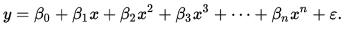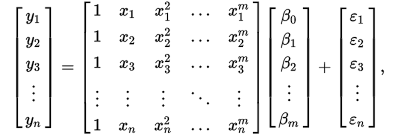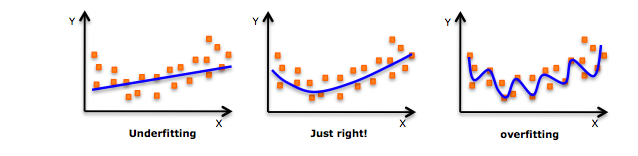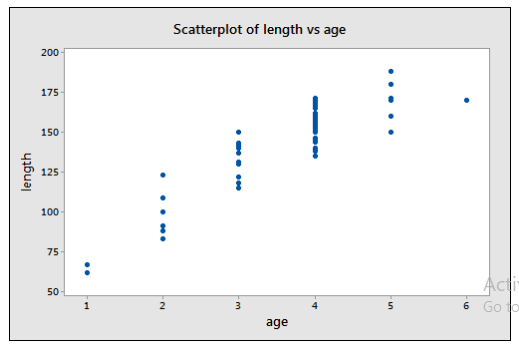# Polynomial Regression

Rating: 4

13470
1. Share:

In this article, we will discuss on another regression model which is nothing but Polynomial regression. Further, how polynomial regression is useful and explained by defining the formula with an example.

Enthusiastic about exploring the skill set of Machine Learning? Then, have a look at the Machine Learning Online Training together with additional knowledge.

## What is Polynomial Regression?

Polynomial regression is a regression algorithm which models the relationship between dependent and the independent variable is modeled such that the dependent variable Y is an nth degree function of the independent variable Y.

The Polynomial regression is also called as multiple linear regression models in ML. Because we add multiple polynomial terms to the multiple linear regression equation, making it a polynomial regression.

• The polynomial regression fits into a non-linear relationship between the value of X and the value of Y.
• It is a linear model with increasing accuracy.
• The dataset used for multiple regression is nonlinear.
• It uses a linear regression model to fit complex data sets of 'nonlinear functions'.
• The Polynomial regression model has been an important source for the development of regression analysis.
• Therefore: In the Polynomial regression, the initial properties are converted to the required degree of Polynomial properties (2,3, .., n) and then modeled by the linear model.### History of Polynomial Regression

It is modeled based on the method of least squares on condition of Gauss Markov theorem. The method was published in 1805 by Legendre and 1809 by Gauss. The first Polynomial regression model came into being in1815 when Gergonne presented it in one of his papers. It is a very common method in scientific study and research.

#### Importance of Polynomial Regression

Polynomial regressions are often the most difficult regressions.

This is niche skill set and is extremely rare to find people with in-depth knowledge of the creation of these regressions

Let us take an example of Polynomial Regression model:

### Polynomial Regression Formula:

The formula of Polynomial Regression is, in this case, is modeled as:Where y is the dependent variable and the betas are the coefficient for different nth powers of the independent variable x starting from 0 to n. The calculation is often done in a matrix form as shown below:This is due to the high amount of data and correlation among each data type. The matrix is always invertible as they follow the statistical rule of m < n and thus become Vandermonde matrix. While it might be tempting to fit the curve and decrease error, it is often required to analyze whether fitting all the points makes sense logically and avoid overfitting. This is a highly important step as Polynomial Regression despite all its benefit is still only a statistical tool and requires human logic and intelligence to decide on right and wrong. Thus, while analytics and regression are great tools to help make decision-making, they are not complete decision makers.

### Polynomial Regression Example

An Polynomial Regression example for overfitting as seen below:It is also advised to keep the order of the polynomial as low as possible to avoid unnecessary complexities. There are two ways of doing a Polynomial regression one is forward selection procedure where we keep on increasing the degree of polynomial till the t-test for the highest order is insignificant. The other process is called backward selection procedure where the highest order polynomial is deleted till the t-test for the higher order polynomial is significant.

An example might be an impact of the increase in temperature on the process of chemical synthesis. Such process is often used by chemical scientists to determine optimum temperature for the chemical synthesis to come into being. Another example might be the relation between the lengths of a bluegill fish compared to its age. Where dependent variable is Y in mm and the dependent variable is X in years.

The marine biologists were primarily interested in knowing how the bluegill fish grows with age and were wanting to determine a correlation between them. The data was collected in the scatter plot given bellow:After complete analysis it was found that the relation was significant and a second order polynomial as shown below –

The coefficient for 0th degree that is the intercept is 13.6, while the coefficients for 1st and 2nd degree is found to be 54.05 and (-) 5.719 respectively.

Conclusion:

So we have gone through a new regression model, i.e. polynomial regression which is widely used in the organizations. This regression model is very difficult to implement and the overall knowledge or the in-depth knowledge of this model is definitely necessary. If you find anything vital that aids to this discussion please key in your suggestions in the comments section below. It will be helpful for rest of the readers who are need of this information.

Regression Related Articles:Stay updated with our newsletter, packed with Tutorials, Interview Questions, How-to's, Tips & Tricks, Latest Trends & Updates, and more ➤ Straight to your inbox!